Click to Chat

1800-1023-196

+91-120-4616500

CART 0

• 0

MY CART (5)

Use Coupon: CART20 and get 20% off on all online Study Material

ITEM
DETAILS
MRP
DISCOUNT
FINAL PRICE
Total Price: Rs.

There are no items in this cart.
Continue Shopping```n- Factor Calculation

Table of Content

Acids

Bases

Related Resources

Acids

Acids are the species which furnish H+ ions when dissolved in a solvent.

For acids, n-factor is defined as the number of H+ ions replaced by 1 mole of acid in a reaction. Note that the n-factor for acid is not equal to its basicity; i.e. the number of moles of replaceable H+ atoms present in one mole of acid.

For example, n-factor of HCI = 1,

n-factor of HNO3 = 1,

n-factor of H2SO4 = 1 or 2, depending upon extent of reaction it undergoes.

H2SO4 + NaOH →  NaHSO4 + H2O

Although one mole of H2SO4 ahs 2 replaceable H atoms but in this reaction H2SO4 has given only one H+ ion, so its n-factor would be 1.

H2SO4 + 2NaOH →  Na2SO4 + 2H2O

The n-factor of H2SO4 in this reaction would be 2.

Similarly,

n-factor of H2SO3 = 1 or 2

n-factor of H2CO3 = 1 or 2

n-factor of H2PO4 = 1 or 2 or 3

n-factor of H3PO3 = 1 or 2 because one of the H is not replaceable in H3PO3. This can be seen using its structure .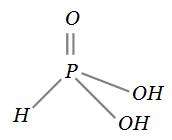The H atoms which are linked to oxygen are replaceable while the H atom linked directly to central atom (P) is nonreplaceable.

n-factor of H3BO3 = 1
In H3BO3, although all three H are linked to oxygen, yet all 3 H are not replaceable. Here, boron atom is electron deficient, so it acts as a Lewis acid. When H3BO3 is added to water, then oxygen atom of H2O through its lone pair attack the boron atom, as follows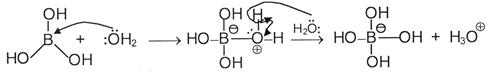The net reaction is H3BO3 + 2H2O →  [B(OH)4]– + H3O+.
Thus, one mole of H3BO3 in solution gives only one mole of H+, so its n-factor is 1.

Bases

Bases are the species, which furnish OH– ions when dissolved in a solvent. For bases, n-factor is defined as the number of OH– ions replaced by 1 mole of base in a reaction. Note that n-factor is not equal to its acidity i.e. the number of moles of replaceable OH– ions present in 1 mole of base.For example,

n-factor of NaOH = 1

n-factor of Zn(OH)2 = 1 or 2

n factor of Ca(OH)2 = 1 or 2

n factor of AI(OH)3 = 1 or 2 or 3

n factor of NH4(OH) = 1

Salts which react such that no atom undergoes change in oxidation state

The n-factor for such salts is defined as the total moles of catioinic/anionic charge replaced in 1 mole of the salt. For the reaction,

2Na3PO4 + 3BaCI2 →  6NaCI + Ba3(PO4)2

To get one mole of Ba3(PO4)2, two moles of Na3PO4 are required, which means six moles of Na+ are completely replaced by 3 moles of Ba2+ ions. So, six moles of cationic charge is replaced by 2 moles of Na3PO4, thus each mole of Na3PO4 replaces 3 moles of cationic charge. Hence, n-factor of Na3PO4 in this reaction is 3.

Salts which react in a manner that only one atom undergoes change in oxidation state and goes only in one product

The n-factor of such salts is defined as the number of moles of electrons exchanged (lost or gained) by one mole of the salt.

Let us have a salt AaBb in which oxidation state of A is +x. It changes to a compound, which has atom D in it. The oxidation state of A in AcD be +y.

A+xa Bb → A+ycD

The n-factor of AaBb is calculated as

n = ½ax – ay½

To calculate n-factor of a salt of such type, we take one mole of the reactant and find the number of mole of the element whose oxidation state is changing. This is multiplied with the oxidation state of the element in the reactant, which gives us the total oxidation state of the element in the reactant. Now, we calculate the total oxidation state of the same element in the product for the same number of mole of atoms of that element in the reactant. Remember that the total oxidation state of the same element in the product is not calculated for the number of mole of atoms of that element in the product.

For example, let us calculate the n-factor KMnO4 for the given chemical change.

KMnO4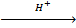Mn+2.

In this reaction, oxidation state of Mn changes from +7 to +2. Thus, KMnO4 is acting as oxidizing agent, since it is reduced.

Hence n-factor of KMnO4 = ½1 × (+7) – 1 × (+2)½ = 5

Similarly,

KMnO4Mn+4

n-factor of KMnO4 = ½1 × (+7) – 1 × (+4)½ = 3

KMnO4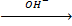Mn+6

n-factor of KMnO4 = ½1 × (+7) – 1 × (+6)½ = 1

It can be seen that in all the above chemical changes, KMnO4 is acting as oxidizing agent, yet its n-factor is not same in all reactions. Thus, the n-factor of a compound is not fixed, it depends on the type and the extent of reaction it undergoes.

Solved Example

Question :

Calculate the n-factor of reactants in the given chemical changes?

K2Cr2O7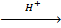Cr3+

C2→  CO2

S2SI– → ICI+6

Solution :

K2Cr2O7Cr3+ The oxidation state of Cr changes from +6 to +3.

Hence n = ½2 × (+6) –2 × (+3)½ = 6

C2→  CO2

Carbon get oxidized from +3 to +4.

Hence n = ½2 × (+3) – 2 × (+4)½ = 2.

S2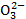CThe oxidation state of sulphur changes from +2 to +6.

Hence n = ½2 × (+2) – 2 × (+6)½ = 8

I– → ICl

I– get oxidized to I+.

Hence n = ½2 × (+3) – 2 × (+4)½ = 2.

Salts that react in a manner that only one atom undergo change in oxidation state but goes in two products with the same oxidation state.
Let us have a salt AaBb in which oxidation state of A is –x. It undergoes a reaction such that element A changes it oxidation state and goes in more than one (two) products with the same oxidation state (but different oxidation state than in the reactant). In such case, the n-factor is calculated in the same manner as in case 4.

Let the chemical change be
A+xaBb → A+ycD + A+yeF

In such cases, the number of products in which element A is present is of no significance since the oxidation state of A in both the products is same. The point of importance is not the number of products containing that element which undergoes change in oxidation state but the oxidation state of the element is of importance. The
n-factor of AaBb is calculated in the same way as in case 4.
Hence n-factor of AaBb = ½ax – ay½
For example, let us calculate the n-factor of K2Cr2O7 for the given chemical change.
Cr2O72– → Cr3+ + Cr3+
In this reaction, oxidation state of Cr changes from +6 to +3 in both products.
Hence n-factor of K2Cr2O7 = ½2 × (+6) –2 × (+3)½ = 6

Salts which react in a manner that only one atom undergoes change in oxidation state but goes in two products with different oxidation state (different than in the reactant) as a result of either oxidation or reduction

Let the chemical change be
A+xaBb → A+ycD + A+yeF

In such cases, n-factor calculation is not possible until we know that how much of A has changed its oxidation state to +y and how much of A has changed its oxidation state to +z. This is because the number of moles of electrons lost or gained by one mole of AaBb would depend on the fact that how much of A underwent change to oxidation state +y and how much of A underwent change to oxidation state +z. This is possible only by knowing the balanced chemical reaction. If we know the balanced chemical reaction, then the n-factor calculation is of no use because problem can be solved using mole concept. But nevertheless, n-factor calculation in such cases can be done as follows.

Let us take a chemical change, 2Mn+7 → Mn+4 + Mn+2 out of the two moles of Mn+7, one mole Mn+7 changes to Mn+4 by gaining 3 mole of electrons and the other mole of Mn+7 changes to Mn+2 by gaining 5 mole of electrons, so in all 8 mole of electrons are gained by 2 mole of Mn+7. So each mole of Mn+7 has gained 8/2 = 4 mole of electrons.
Thus, 4 would be the n-factor of Mn+7 in this reaction.

If the reaction would have been
3Mn+7 → 2Mn+2 + Mn+4
Out of 3 moles of Mn+7, two moles of Mn+7 changes to Mn+2 by gaining 10 mole of electrons and one mole of Mn+7 changes to Mn+4 bygained 3 mole of electrons.
Thus each mole of Mn+7 have gained 13/3 mole of electrons. Therefore, the n-factor of Mn+7 in this reaction would be 13/3.Note that n-factor can be fraction because it is not the number of electrons exchanged but it is the number of moles of electrons exchanged which can be a fraction.

Now, if the reaction would have been 3Mn+7 →  Mn+2 + 2Mn+4. Thus, each mole of Mn+2.

Thus, each mole of Mn+7 have gained 11/3 mole of electron. Therefore, n-factor of Mn+7 in this reaction would be 11/3.

Salts which react in a fashion that only one atom undergoes change in oxidation state but goes in two products with different oxidation state (in one product with same oxidation state and in other with different oxidation state than in the reactant)

Let the reaction be

A+xaBb → A+xeF + A+ycD

For such reactions also, the n-factor calculation is not possible without the knowledge of balanced chemical reaction because n-factor of AaBb would depend on the fact that how much of A underwent change to oxidation state +y and how much of A remained in the same oxidation state +x.
For example, if we have a chemical change as 2Mn+7 → Mn+7 + Mn+2 (the compounds containing Mn in +7 state in reactant and product are different.
In this reaction, 5 moles of electrons are gained by 2 moles of Mn+7, so each mole of Mn+7 takes up 5/2 mole of electrons.
Therefore, n-factor of Mn+7 in this reaction would be 5/2.
Calculate n-factor of Mn+7, if the reaction would have been 3Mn+7 → Mn+7 + 2Mn+2.

Salts that react in a manner that two type of atoms in the salt undergo change in oxidation state (both the atoms are either getting oxidised or reduced).

Let the change be represented as
A+xaBb → A+xeF + A+ycD

In this reaction, both A and B are changing their oxidation states and both of them are either getting oxidized or reduced. In such case, the n-factor of the compound would be the sum of individual n-factors of A and B.
n-factor of A = ½ax – ay½
n-factor of B = ½– ax – bx½ because the total oxidation state of ‘b’ B’s in the reactant is –ax (as the total oxidation state of ‘a’ A’s in the reactant is +ax) and the total oxidation state of y B’s in the product is bz.
Hence n-factor of AaBb = ½ax – ay½ + ½– ax – bz½
In general, the n-factor of the salt will be the total number of mole of electrons lost or gained by one mole of the salt.
For example, we have a reaction Cu+12S–2 →  Cu2+ + SO2 in which Cu+ and S2– both are getting oxidized to Cu2+ and S+4 respectively.
Hence n-factor of Cu2S = ½2 × (+1) –2 (+2)½ + ½1 × (–2) –1 × (+4)½ = 8

Refer to the following video for a solved problem on normality and n– factor calculation.

Salts that react in a manner that two atoms in the salt undergoes change in oxidation state (on atom is getting oxidised and the other is getting reduced).
If we have a salt which react in a fashion that atoms of one of the elements are getting oxidized and the atoms of another element are getting reduced and no other element on the reactant side is getting oxidized or reduced, than the n-factor of such a salt can be calculated either by taking the total number of moles of electrons lost or total number of mole of electrons gained by one mole of the salt.
For example, decomposition reaction of KCIO3 is represented as
KCIO3 → KCI + O2
In this reaction, O2– is getting oxidized to O2 and CI+5 is getting reduced to CI–1. In each case, 6 mole of electrons are exchanged whether we consider oxidation or reduction.
n-factor of KCIO3 considering oxidation = ½3(–2)–3(0)½ = 6
or n-factor of KCIO3 considering reduction = ½1 × (+5) –1 × (–1)½ = 6Slats or compounds which undergoes disproportionation reaction.
Disproportionation reactions are the reactions in which oxidizing and reducing agents are same or the same element from the same compound is getting oxidized as well as reduced. N-factor of a disproportionation reaction can only be calculated using a balanced chemical reaction. We will categorize disproportionation reactions into two types.

Disproportionation reactions in which moles of compound getting oxidized and reduced are same i.e. moles of oxidizing agent and reducing agent are same. The n-factor for such compounds is calculated by either the number of mole of electrons lost or gained by one mole of the compound because in such a case,
n-factor of the compound acting as oxidizing agent or as reducing agent would be same.
For example, 2H2O2 → 2H2O + O2
Out of the 2 mole of H2O2 used in reaction, one mole of H2O2 gets oxidized to O2 (oxidation state of O changes from –1 to 0) while the other mole of H2O2 gets reduced to H2O (oxidation state of O changes from –1 to –2). When 1 mole of H2O2 gets oxidized to O2, the half-reaction would be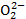→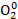+ 2e– and when 1 mole of H2O2 gets reduced to H2O, the half-reaction would be→ 2e– → 2O2–.
Thus, it is evident that one mole of H2O2 (which is either getting oxidized or reduced) will lose or gain 2 mole of electrons. Therefore, n-factor of H2O2 as oxidizing as well as reducing agent in this reaction is 2.
where, H2O2 is not distinguished as how much of it functions as oxidizing agent and how much as reducing agent, then n-factor calculation can be done in the following manner. Find the number of electrons exchanged (lost or gained) using the balanced equation and divide it by the number of moles of H2O2 involved in the reaction. Thus, the n-factor of H2O2 when the reaction is written without segregating oxidizing and reducing agent is= 1.

2H2O2 → 2H2O + O2

(n=1)        (n=1)          (n=2)

Disproportionation reactions in which moles of compound getting oxidized and reduced are not same i.e. moles of oxidizing agent and reducing agent are not same.
6Br2 + 12 OH– → 10Br– + 2BrO–3 + 6H2O
In this reaction, the mole of electrons lost by the oxidation of some of the moles of Br2 are same as the number of moles of electrons gained by the reduction of rest of the moles of Br2. Of the 6 moles of Br2 used, one mole is getting oxidized, loosing 10 electrons (as reducing agent) and 5 moles of Br2 are getting reduced and accepts 10 moles of electrons (as oxidizing agent).
where Br2 is not distinguished as how much of it functions as oxidizing agent and how much as reducing agent, then for calculating n-factor of compounds in such reactions, first find the total number of mole of electrons exchanged (lost or gained) using the balanced equation and divide it with the number of mole of Br2 involved in the reaction to get the number of mole of electrons exchanged by one mole of Br2.
In the overall reaction, the number of mole of electrons exchanged (lost or gained) is 10 and the moles of Br2 used in the reaction are 6. Thus, each mole of Br2 has exchanged 10/6 or 5/3 mole of electrons.
Therefore, the n-factor of Br2 when the reaction is written without segregating oxidizing and reducing agent is 5/3.
6Br2 → 10Br– + 2Br+5
(n=5/3)     (n=1)     (n=5)Question 1: n-factor for  HNO3 is

a. 1

b. 2

c. 3

d. 4

Question 2:  What of the following alternatives does not represents the possible value of n-factor of H2PO4 ?

a. 1

b. 2

c. 3

d. 4

Question 3:  In the reaction 2Na3PO4 + 3BaCI2 → 6NaCI + Ba3(PO4)2

n-factor of Na3PO4 in this reaction is..

a. 1

b. 2

c. 3

d. 4

Question 4:  In the reaction 6Br2 → 10Br– + 2Br+5  the n-factor of Br2 is

a. 1

b. 2

c. 3

d. None of the aboveQ.1

Q.2

Q.3

Q.4

a

d

c

d

Related Resources

Have a look  syllabus of chemistry for  IIT JEE

You can also refer to volumetric analysis

```### Course Features

• 731 Video Lectures
• Revision Notes
• Previous Year Papers
• Mind Map
• Study Planner
• NCERT Solutions
• Discussion Forum
• Test paper with Video Solution